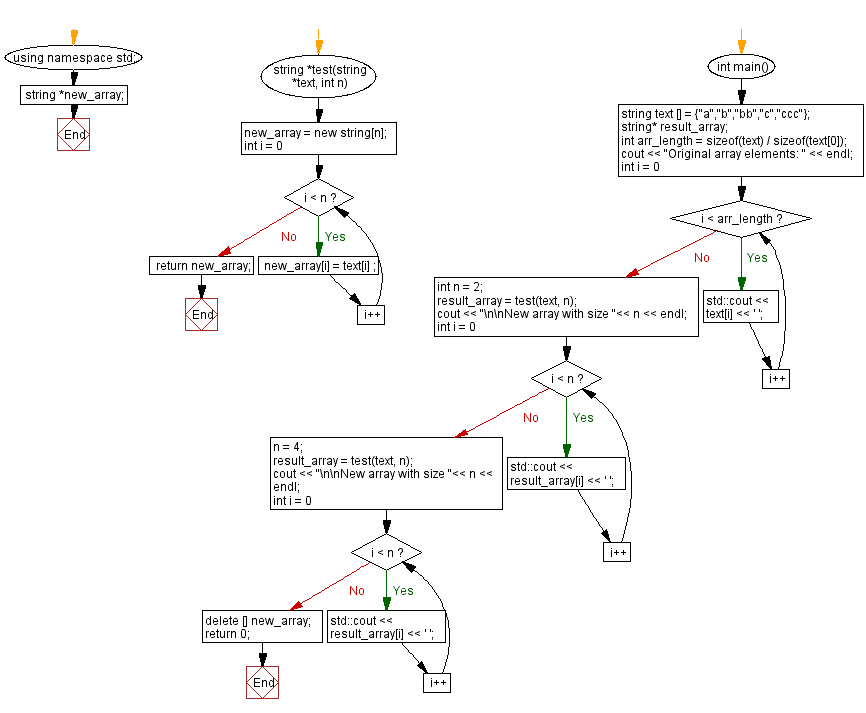﻿ CPP - New array from an existing array

# C++ Exercises: New array from an existing array

## C++ Basic Algorithm: Exercise-120 with Solution

Write a C++ program to create a new array using the first n strings from a given array of strings. (n>=1 and <=length of the array).

Test Data:
({"a", "b", "bb", "c", "ccc" }, 2) -> {"a", "b"}
({"a", "b", "bb", "c", "ccc" }, 3) -> {"a", "b", "bb"}

Sample Solution:

C++ Code :

``````#include <iostream>

#include<string.h>

using namespace std;
string *new_array;
string *test(string *text, int n)
{
new_array = new string[n];
for(int i = 0; i < n; i++)
{
new_array[i] = text[i] ;
}
return new_array;
}
int main(){
string text [] = {"a","b","bb","c","ccc"};
string* result_array;
int arr_length = sizeof(text) / sizeof(text);
cout << "Original array elements: " << endl;
for (int i = 0; i < arr_length; i++)
{
std::cout << text[i] << ' ';
}
int n = 2;
result_array = test(text, n);
cout << "\n\nNew array with size "<< n << endl;
for(int i = 0; i < n; i++)
{
std::cout << result_array[i] << ' ';
}
n = 4;
result_array = test(text, n);
cout << "\n\nNew array with size "<< n << endl;
for(int i = 0; i < n; i++)
{
std::cout << result_array[i] << ' ';
}
delete [] new_array;
return 0;
}
``````

Sample Output:

```Original array elements:
a b bb c ccc

New array with size 2
a b

New array with size 4
a b bb c
```

Flowchart:C++ Code Editor:

What is the difficulty level of this exercise?

﻿

## C++ Programming: Tips of the Day

What is the usefulness of `enable_shared_from_this?

It enables you to get a valid shared_ptr instance to this, when all you have is this. Without it, you would have no way of getting a shared_ptr to this, unless you already had one as a member.

```class Y: public enable_shared_from_this
{
public:

shared_ptr f()
{
return shared_from_this();
}
}

int main()
{
shared_ptr p(new Y);
shared_ptr q = p->f();
assert(p == q);
assert(!(p < q || q < p)); // p and q must share ownership
}
```

The method f() returns a valid shared_ptr, even though it had no member instance. Note that you cannot simply do this:

```class Y: public enable_shared_from_this
{
public:

shared_ptr f()
{
return shared_ptr(this);
}
}
```

The shared pointer that this returned will have a different reference count from the "proper" one, and one of them will end up losing and holding a dangling reference when the object is deleted.

Ref : https://bit.ly/3pwVzzz